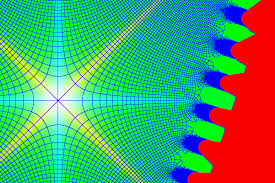Statistical physics is a branch of physics that uses methods of probability theory and statistics, and particularly the mathematical tools for dealing with large populations and approximations, in solving physical problems

## Level## IntroductionStatistical physics is a branch of physics that uses methods of probability theory and statistics, and particularly the mathematical tools for dealing with large populations and approximations, in solving physical problems. It can describe a wide variety of fields with an inherently stochastic nature. Its applications include many problems in the fields of physics, biologychemistryneurology, and even some social sciences, such as sociology. Its main purpose is to clarify the properties of matter in aggregate, in terms of physical laws governing atomic motion.

In particular, statistical mechanics develops the phenomenological results of thermodynamics from a probabilistic examination of the underlying microscopic systems. Historically, one of the first topics in physics where statistical methods were applied was the field of mechanics, which is concerned with the motion of particles or objects when subjected to a force.